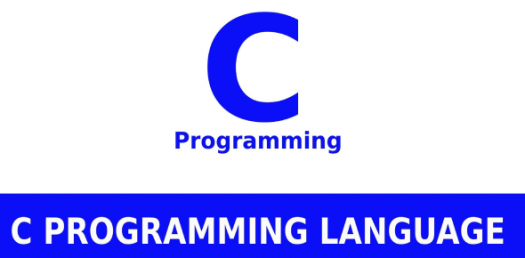# Can You Pass This C Programming Language Test?

10 Questions | Total Attempts: 200SettingsCan you pass this C programming language test? If you are looking to see just how well you understand this language, then taking this quiz will give you an idea of how much more work you need to put in to understand it. Give it a try and get to refresh your memory in less than ten minutes. All the best!

• 1.
#include int main(){     int i=2,j=2;     while(i+1?--i:j++)          printf("%d",i);     return 0; }
• A.

1

• B.

3

• C.

2

• 2.
#include int main(){     static int i;     for(++i;++i;++i) {          printf("%d ",i);          if(i==4) break;     }     return 0; }
• A.

36

• B.

24

• C.

1

• 3.
#include int main(){     for(;;) {          printf("%d ",10);     }     return 0; }
• A.

Infinite loop

• B.

10

• C.

Will not work

• 4.
#include int r(); int main(){     for(r();r();r()) {          printf("%d ",r());     }     return 0; } int r(){     int static num=7;     return num--; }
• A.

4 7

• B.

5 2

• C.

4 1

• 5.
Note:  Answer of all question is based upon that compilers whose word size is two byt#include void main(){     printf("%d\t",sizeof(6.5));     printf("%d\t",sizeof(90000));     printf("%d",sizeof('A')); }
• A.

4 2 1

• B.

4 4 1

• C.

8 4 2

• 6.
#include void main(){     double num=5.2;     int  var=5;     printf("%d\t",sizeof(!num));     printf("%d\t",sizeof(var=15/2));     printf("%d",var); }
• A.

4 2 7

• B.

2 2 5

• C.

2 4 7

• 7.
#include void main(){     int a=5,b=10,c=1;     if(a&&b>c){          printf("cquestionbank");     }     else{          break;     } }
• A.

Cquestionbank

• B.

Run time error

• C.

Compilation error

• 8.
#include void main(){     int a=100;     if(a>10)          printf("M.S. Dhoni");     else if(a>20)          printf("M.E.K Hussey");     else if(a>30)            printf("A.B. de villiers"); }
• A.

M.S. Dhoni

• B.

A.B. de villiers

• C.

M.S Dhoni M.E.K Hussey A.B. de Villiers

• 9.
#include void main(){     int x=-1,y=-1;     if(++x=++y)          printf("R.T. Ponting");     else          printf("C.H. Gayle"); }
• A.

R.T Ponting

• B.

Compilation error

• C.

Warning: Condition is always true

• 10.
#include void main(){     int m=5,n=10,q=20;     if(q/n*m)          printf("William Gates");     else          printf(" Warren Buffet");          printf(" Carlos Slim Helu"); }
• A.

William Gates

• B.

Warren Buffet Carlos Slim Helu

• C.

None of the above

Related TopicsBack to top